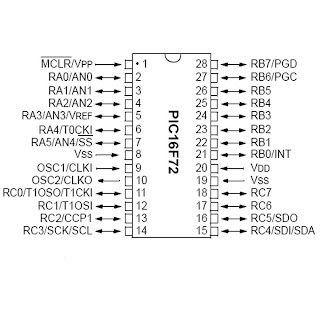## Friday, June 15, 2007

### A digital clock using PIC16F72

'****************************************************************
'* Name : clock.BAS * '* *
'* Author : [RAVEENDRA PAI G] *
'* Notice : Copyright (c) 2007 [RAVEENDRA PAI G] *
'* Version : 1.0 *
'* Notes :digital clock *
: The program counts if the portb.7=0 and loads the count if the portb.7=1 following are the functions of the ports
portA=hour indicator in bcd
portc=second indicator in bcd last pin ie pin7 is not used for indicating time its for am or pm
portb=min indicator with last pin i/p
NOTE that the statements starting with ' are comments!
'****************************************************************
b0 var byte 'b0 as byte
b1 var byte
b2 var byte
b3 var byte

b0=b1=0 'initialization
b2=1
l6: 'redefinition label
TRISA=0
TRISB=128 'making the pins i/p as well as o/p
TRISC=0

l1: 'label1

poke PORTA,b2 'writing variable b2 to portA
POKE PORTB,b1
POKE PORTC,b0
pause 1000 'pause for 1 sec

peek PORTB,b3 'peeking the portB for some interrupts on the i/p pin 7
if ((b3 AND 128)=128) then goto l5 'checking the pin condition

b0=b0+1 'updating the sec variable
if (b0 AND 10)=10 then b0=b0+6 'if b0 value is (lsb)10 then its is updated to bcd
if (b0=96) then goto l2 'for 60 it is updated to 0 and min =min+1
l9:
goto l1
l2:
b0=0
b1=b1+1
if ((b1 AND 10)=10) then b1=b1+6 'same function as b0 updation but min
if ((b1=96) or (b1=224)) then goto l3 'msb is the am or pm indicator
l8:
goto l9

l3:

b1=0
b2=b2+1 'hour update
if ((b2 AND 10)=10) then b2=b2+6
if (b2=19) then goto l4 'hour returns to 0 and am<=>pm
l10:
goto l8
l4:
if ((b0 AND 128)=0) then b0=0
if ((b0 AND 128)=128) then b0=128
b1=0
b2=1
goto l10
l5: 'interrupt loop for time reset
TRISA=31 'redefining the pins all as i/p pins
TRISB=255
TRISC=255
peek PORTC,b0 'peeking pins and writing them on variables
PEEK PORTB,b1
PEEK PORTA,b2
if ((b1 AND 128)=128) then goto l6 'check for interrupt cancel portb.7=0
goto l5
#########################################################
for the bcd to sevensegment conversion use a 74LS48 :
PIC16F72connect the memoryclr complement to vcc through a resistor(4.7k)
connect the vdd to vcc
connect the vss to gnd
connect the 10 and 9 to a common point through a capacitor of value 22pf (10 to 33pf)and connect that point to vcc through a .1microfar capacitor
Use switch to load the time and count !